# Math in Focus Grade 3 Chapter 11 Practice 4 Answer Key Liters and Milliliters

Practice the problems of Math in Focus Grade 3 Workbook Answer Key Chapter 11 Practice 4 Liters and Milliliters to score better marks in the exam.

## Math in Focus Grade 3 Chapter 11 Practice 4 Answer Key Liters and Milliliters

Find the volume of water in each measuring cup.

Question 1.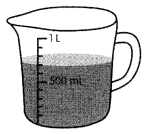____________ mL
700 ml
Explanation:
The volume of water in the cup shows at 700 ml
Each point value = 100 ml

Question 2.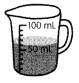____________ mL
10 ml
Explanation:
The volume of water in the cup shows at 60 ml
Each point value = 10 ml

Question 3.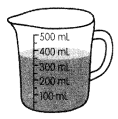___________ mL
350 ml
Explanation:
The volume of water in the cup shows at 350 ml
Each point value = 100 ml

The water from each container is poured into measuring cups. Find the capacity of each container.

Question 4.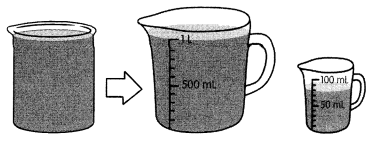__________ L __________ mL
1 L 80 ML
Explanation:
The water from each container is poured into measuring cups.
the capacity of each container is indicated as 1 l 80 ml

Question 5.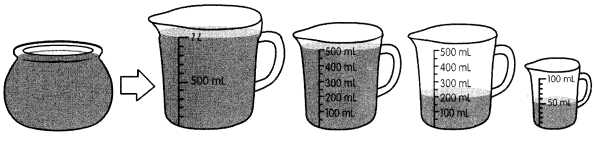__________ L __________ mL
1 L 750 ML
Explanation:
The water from each container is poured into measuring cups.
the capacity of each container is indicated as 1 L +  500 ML +  200 ML + 50 ML =  1 L 750 ML

Complete.

A teacher filled Containers A and B completely with water. However, he had only enough water left to fill $$\frac{1}{2}$$ of Container C. Find the volume of water in each container and the capacity of each container.

Question 6.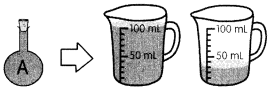Volume of water in Container A = 130 mL
Capacity of Container A = 130 mL
Volume of water in Container A = 100 mL
100 ML + 30 ML = 130 ML
Capacity of Container A = 100 mL
Explanation:
The water from each container is poured into measuring cups.
the capacity of each container is indicated as 180 ML
capacity of  A = 130 ML
capacity of  B = 100 ML Occupied full
C = 100 ML Occupied 30 ML

Question 7.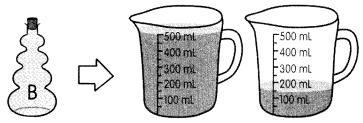Volume of water in Container B = __________ mL
Capacity of Container B = __________ mL
Volume of water in Container B = 650 mL
Capacity of Container B = 150 mL
Explanation:
The water from each container is poured into measuring cups.
the capacity of each container is indicated as 650 ml
Container B can hold 500 + 150 mL = 650 mL

Question 8.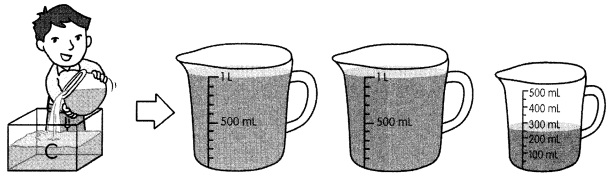Volume of water in Container C = __________ L __________ mL
Capacity of Container C = __________L __________ mL
Volume of water in Container C = 2 L 250 mL
Capacity of Container C = 2 L 250 mL
Explanation:
The water from each container is poured into measuring cups.
the capacity of each container is indicated as 2 L 250 ML

Complete.

Question 9.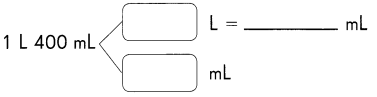1 L 400 mL = __________ mL + __________ mL
= __________ mL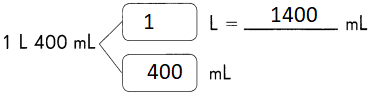1 L 400 mL = 1000 mL + 400 mL
= 1400 mL
Explanation:
Converting Liters to milliliters
1 Liter = 1000 milliliters

Question 10.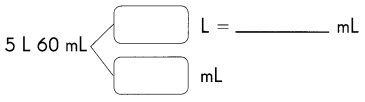5 L 60 mL = __________ mL + __________ mL
= __________ mL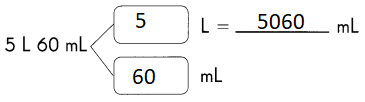5 L 60 mL = 5000 mL + 60 mL
= 5060 mL
Explanation:
Converting Liters to milliliters
1 Liter = 1000 milliliters

Write in milliliters.

Question 11.
2 L 450 mL = __________ mL + __________ mL = __________ mL
2 L 450 mL = 2000 mL + 450 mL = 2450 mL
Explanation:
Converting Liters to milliliters
1 Liter = 1000 milliliters

Question 12.
1 L 105 mL = __________ mL + __________ mL = __________ mL
1 L 105 mL = 1000 mL + 105 mL = 1105 mL
Explanation:
Converting Liters to milliliters
1 Liter = 1000 milliliters

Question 13.
2 L 45 mL = __________ mL + __________ mL = __________ mL
2 L 45 mL = 2000 mL + 45 mL = 2045 mL
Explanation:
Converting Liters to milliliters
1 Liter = 1000 milliliters

Question 14.
3 L 9 mL = __________ mL + __________ mL = __________ mL
3 L 9 mL = 3000 mL + 9 mL = 3009 mL
Explanation:
Converting Liters to milliliters
1 Liter = 1000 milliliters

Complete.

Question 15.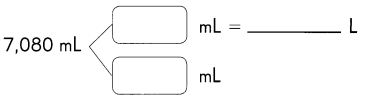7,080 mL = ___________ L __________ mL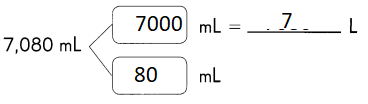7,080 mL = 7 L 80 mL
Explanation:
Converting given  milliliters to the liters and milliliters
1 Liter = 1000 milliliters

Question 16.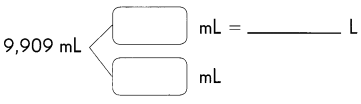9,909 mL = ___________ L __________ mL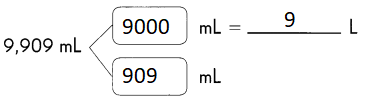9,909 mL = 9 L 909 mL
Explanation:
Converting given  milliliters to the liters and milliliters
1 Liter = 1000 milliliters

Write in liters and milliliters.

Question 17.
4,900 mL = ___________ mL + ___________ mL = ___________ L ___________ mL
4,900 mL = 4000 mL + 900 mL = 4 L 900 mL
Explanation:
Converting given  milliliters to the liters and milliliters
1 Liter = 1000 milliliters

Question 18.
6,505 mL = ___________ mL + ___________ mL = ___________ L ___________ mL
6,505 mL = 6000 mL + 505 mL = 6 L 505 mL
Explanation:
Converting given  milliliters to the liters and milliliters
1 Liter = 1000 milliliters

Question 19.
2,090 mL = ___________ mL + ___________ mL = ___________ L ___________ mL
2,090 mL = 2000 mL + 90 mL = 2 L 90 mL
Explanation:
Converting given  milliliters to the liters and milliliters
1 Liter = 1000 milliliters

Question 20.
2,005 mL = ___________ mL + ___________ mL = ___________ L ___________ mL
2,005 mL = 2000 mL + 5 mL = 2 L 5 mL
Explanation:
Converting given  milliliters to the liters and milliliters
1 Liter = 1000 milliliters

Choose the unit you would use to measure each. Write liters or milliliters.

Question 21.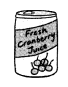A can of cranberry juice is about 300 ___________
A can of cranberry juice is 300 Milliliters
Explanation:
A can of cranberry juice is 300 Milliliters
Because the can of juice is very small
the volume will be less

Question 22.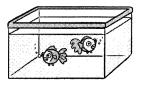The volume of water in a fish tank is about 10 ___________.